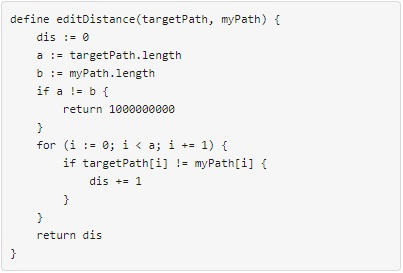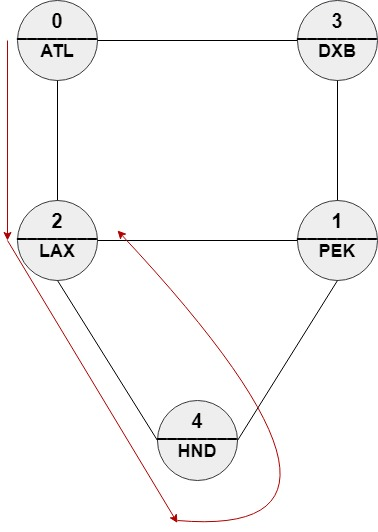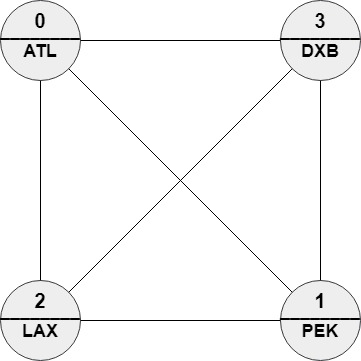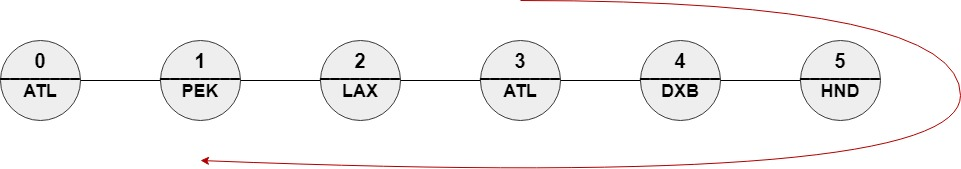Formatted question description: https://leetcode.ca/all/1548.html

# 1548. The Most Similar Path in a Graph

Hard

## Description

We have n cities and m bi-directional roads where roads[i] = [a_i, b_i] connects city a_i with city b_i. Each city has a name consisting of exactly 3 upper-case English letters given in the string array names. Starting at any city x, you can reach any city y where y != x (i.e. the cities and the roads are forming an undirected connected graph).

You will be given a string array targetPath. You should find a path in the graph of the same length and with the minimum edit distance to targetPath.

You need to return the order of the nodes in the path with the minimum edit distance. The path should be of the same length of targetPath and should be valid (i.e. there should be a direct road between ans[i] and ans[i + 1]). If there are multiple answers return any one of them.

The edit distance is defined as follows:Follow-up: If each node can be visited only once in the path, What should you change in your solution?

Example 1:Input: n = 5, roads = [[0,2],[0,3],[1,2],[1,3],[1,4],[2,4]], names = [“ATL”,”PEK”,”LAX”,”DXB”,”HND”], targetPath = [“ATL”,”DXB”,”HND”,”LAX”]

Output: [0,2,4,2]

Explanation: [0,2,4,2], [0,3,0,2] and [0,3,1,2] are accepted answers.

[0,2,4,2] is equivalent to [“ATL”,”LAX”,”HND”,”LAX”] which has edit distance = 1 with targetPath.

[0,3,0,2] is equivalent to [“ATL”,”DXB”,”ATL”,”LAX”] which has edit distance = 1 with targetPath.

[0,3,1,2] is equivalent to [“ATL”,”DXB”,”PEK”,”LAX”] which has edit distance = 1 with targetPath.

Example 2:Input: n = 4, roads = [[1,0],[2,0],[3,0],[2,1],[3,1],[3,2]], names = [“ATL”,”PEK”,”LAX”,”DXB”], targetPath = [“ABC”,”DEF”,”GHI”,”JKL”,”MNO”,”PQR”,”STU”,”VWX”]

Output: [0,1,0,1,0,1,0,1]

Explanation: Any path in this graph has edit distance = 8 with targetPath.

Example 3:Input: n = 6, roads = [[0,1],[1,2],[2,3],[3,4],[4,5]], names = [“ATL”,”PEK”,”LAX”,”ATL”,”DXB”,”HND”], targetPath = [“ATL”,”DXB”,”HND”,”DXB”,”ATL”,”LAX”,”PEK”]

Output: [3,4,5,4,3,2,1]

Explanation: [3,4,5,4,3,2,1] is the only path with edit distance = 0 with targetPath.

It’s equivalent to [“ATL”,”DXB”,”HND”,”DXB”,”ATL”,”LAX”,”PEK”]

Constraints:

• 2 <= n <= 100
• m == roads.length
• n - 1 <= m <= (n * (n - 1) / 2)
• 0 <= a_i, b_i <= n - 1
• a_i != b_i
• The graph is guaranteed to be connected and each pair of nodes may have at most one direct road.
• names.length == n
• names[i].length == 3
• names[i] consists of upper-case English letters.
• There can be two cities with the same name.
• 1 <= targetPath.length <= 100
• targetPath[i].length == 3
• targetPath[i] consists of upper-case English letters.

## Solution

First, use a map to store each city’s all adjacent cities. Then use dynamic programming. Create a 2D array dp of t rows and n columns, where t is the length of targetPath and n is the number of cities, and dp[i][j] represents the minimum edit distance of the path with length i + 1 where the last city is j. Initialize all elements in dp to infinity. For each element in dp, the value of dp[i] is 0 if names[i] equals targetPath, or 1 otherwise. Create another 2D array paths of t rows and n columns, where paths[i][j] represents the previous city of city j when the j-th city is at the i-th index. Initialize all elements of paths to -1.

For i from 1 to t - 1 and j from 0 to n - 1, if dp[i - 1][j] is not infinity, then obtain all the adjacent cities of city j, and for each adjacent city k, calculate the value of dp[i][k], and set paths[i][k] = j.

After all values in dp and paths are calculated, find the minimum value of dp[t - 1] and the corresponding index minIndex. Starting from minIndex, obtain the path with the minimum edit distance using paths. Finally, return the path.

class Solution {
public List<Integer> mostSimilar(int n, int[][] roads, String[] names, String[] targetPath) {
Map<Integer, List<Integer>> roadMap = new HashMap<Integer, List<Integer>>();
int city0 = road, city1 = road;
List<Integer> list0 = roadMap.getOrDefault(city0, new ArrayList<Integer>());
List<Integer> list1 = roadMap.getOrDefault(city1, new ArrayList<Integer>());
}
int pathCount = targetPath.length;
int[][] dp = new int[pathCount][n];
for (int i = 0; i < pathCount; i++)
Arrays.fill(dp[i], Integer.MAX_VALUE);
int[][] paths = new int[pathCount][n];
Arrays.fill(paths, -1);
for (int i = 0; i < n; i++)
dp[i] = names[i].equals(targetPath) ? 0 : 1;
for (int i = 1; i < pathCount; i++) {
for (int j = 0; j < n; j++) {
if (dp[i - 1][j] == Integer.MAX_VALUE)
continue;
List<Integer> list = roadMap.getOrDefault(j, new ArrayList<Integer>());
for (int k : list) {
if (dp[i][k] > dp[i - 1][j] + (names[k].equals(targetPath[i]) ? 0 : 1)) {
dp[i][k] = dp[i - 1][j] + (names[k].equals(targetPath[i]) ? 0 : 1);
paths[i][k] = j;
}
}
}
}
int minDistance = Integer.MAX_VALUE, minIndex = -1;
for (int i = 0; i < n; i++) {
if (minDistance > dp[pathCount - 1][i]) {
minDistance = dp[pathCount - 1][i];
minIndex = i;
}
}
List<Integer> mostSimilarPath = new ArrayList<Integer>();
for (int i = pathCount - 1; i >= 0; i--) {